### Home > CALC > Chapter Ch10 > Lesson 10.1.8 > Problem10-84

10-84.
1. Examine the graph of f(t) shown below. Homework Help ✎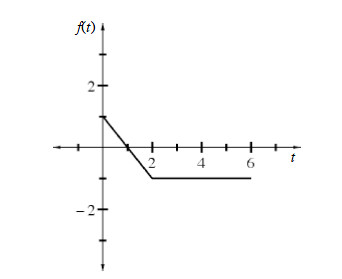Let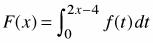.

1. What is the domain of F(x) ?

2. For what values of x does F(x) attain its absolute maximum and minimum? Justify your answer.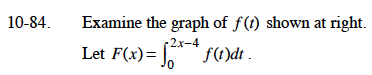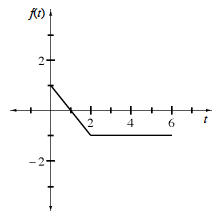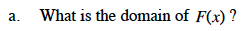f(t) is defined for 0 ≤ t ≤ 6, so 0 ≤ 2x – 4 ≤ 6.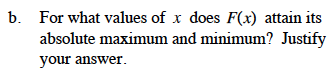F(x) represents the area under the curve. Review the graph.
For what value of t will this area be a maximum? A minimum?

The maximum occurs when t = 1. The minimum occurs when t = 6.
What are the corresponding x-values?